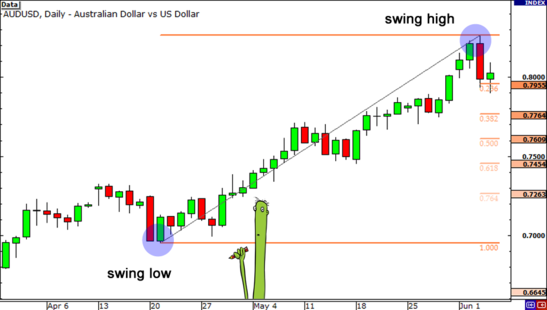Partner Center Find a Broker

Fibonacci retracement (or Fib retracement) is a tool used by technical analysts to identify key support and resistance levels.

The support and resistance levels are plotted as horizontal lines and used to estimate likely reversal points during an uptrend or downtrend.

They do so by using Fibonacci ratios as percentages.The Fib retracement tool is derived from a string of numbers identified by mathematician Leonardo Fibonacci in the 13th century.

This string is called the Fibonacci sequence.

Certain mathematical relationships between numbers in this sequence create ratios that are then plotted to a chart. These ratios are:

• 0%
• 23.6%
• 38.2%
• 61.8%
• 78.6%
• 100%

The above levels are seen as significant levels in where price may bounce back from or accelerate towards.

These levels can be used to identify key support and resistance levels which can be useful for planning entry or exit points (such as stop loss placement) for your trades.

While technically not a Fibonacci ratio, some traders also consider the 50% level to have some significance, as it represents the midpoint of the price range.

Fibonacci ratios outside of the 0-100% range may also be used, such as:

• 161.8%
• 261.8%
• 423.6%

## How to use Fibonacci Retracement

Typically, the tool is drawn by picking two extreme points within the price range, such as a high and a low.

This range is then used as the basis for further analysis.

Usually, the tool is used for mapping out levels inside of the range, but it may also provide insights into important price levels outside of the range.

Typically, this range is drawn according to the underlying trend.

So, in an uptrend, the low point would be the 1 (or 100%), while the high point would be 0 (0%).

By drawing Fib retracement lines over an uptrend, traders can get an idea of potential support levels that may be tested in case the market starts to retrace – hence the term retracement.

Conversely, during a downtrend, the low point would be 0 (0%), and the high point 1 (100%).

The retracement, in this case, refers to the movement from the bottom (a bounce).

In this context, the Fibonacci retracement tool may provide insights into potential resistance levels if the market starts to move up.

## How to Trade Fibonacci Levels

Using the Fibonacci retracement tool isn’t useful for determining the overall trend in price but can help to predict levels of support and resistance within a large trend reversal.

Traders may use Fibonacci levels to determine potential entry points, stop loss levels, and take profit levels.

This can vary significantly depending on each trader’s setup, strategy, and trading style.

Some strategies involve profiting on the range between two specific Fibonacci levels.

For example, consider an uptrend followed by a retracement. Buying at the 38.2% retracement level then selling at the 23.6% level could be an interesting strategy.

Fibonacci levels are also often combined with the Elliott Wave Theory to find correlations between wave structures and potential areas of interest.

This can be a powerful strategy to predict the extent of retracements in different waves of a particular market structure.

## Fibonacci is Like Santa Clause

Fibonacci numbers are found everywhere in nature, and many traders believe that they have relevance when charting financial markets.

However, as with all technical indicators, the relationship between price action, chart patterns, and indicators are NOT based on any scientific principle or physical law.

The Fibonacci retracement tool’s usefulness is dependant on the number of market participants paying attention to it.

The more people looking at the same Fibonacci levels, the stronger its predictive power.

These levels are created by picking two extreme points in a chart’s pattern and then dividing the vertical distance by the key levels predominantly used in trading of 23.6%, 38.2%, 50%, 61.8%, 78.6% and 100%.

Markets move in rhythms. An impulse wave that defines a major market trend will have a corrective wave before the next impulse wave reaches new territory.

This occurs in either bull market or bear market conditions. The most common approach to working with corrections is to relate the size of a correction to a percentage of a prior impulsive market move.

In regards to the 3-wave patterns, Fibonacci Retracement indicates how far a corrective wave B could go before wave C is born.

The first support level is the one marked by 38.2% and if price moves through it then it becomes a resistance line and a new support level shifts to Fibonacci level of 61.8%.

Fibonacci retracements are one of the four types of Fibonacci studies used for predicting levels of support and resistance.

Fibonacci retracements are used immediately after a strong price movement either up or down. An imaginary vertical line is drawn across the chart between two extreme price values, one high and one low.

Then a number of horizontal lines are drawn perpendicular to the imaginary vertical at significant Fibonacci values.

The most common number of lines is five, drawn at 0%, 38.2%, 50%, 61.8%, and 100% of the length of the line (starting from either end), but some traders have been known to use even more retracement lines than this.

Following a strong price movement in either direction, markets tend to “retrace” much of their change in price, and the levels at which this retracement reverses or pauses often correspond with the horizontal lines on the Fibonacci retracement chart.